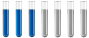## Chemistry the rate of reactions

Chemistry and homework help forum.

Organic Chemistry, Analytical Chemistry, Biochemistry, Physical Chemistry, Computational Chemistry, Theoretical Chemistry, High School Chemistry, Colledge Chemistry and University Chemistry Forum.

Share your chemistry ideas, discuss chemical problems, ask for help with scientific chemistry questions, inspire others by your chemistry vision!

Please feel free to start a scientific chemistry discussion here!

Discuss chemistry homework problems with experts!

Ask for help with chemical questions and help others with your chemistry knowledge!

Moderators: Xen, expert, ChenBeier

taylorswift08
Full MemberPosts: 11
Joined: Mon Mar 20, 2023 8:36 am

### Chemistry the rate of reactions

Under experimental conditions, at a temperature of 320°C, one mole of NOBr decomposes according to the reaction NOBr= NO +1/2 Br2. The coefficients before the formulas of the substances in the reaction are simultaneously the values ​​of powers of their concentrations. Calculate how many moles of NOBr are left unreacted if it is heated for 5 hours and the rate constant is 2.2* 10^-5 mol^¹* s^-1
ChenBeier
Distinguished MemberPosts: 1308
Joined: Wed Sep 27, 2017 7:25 am
Location: Berlin, Germany

### Re: Chemistry the rate of reactions

Calculate the moles consumed = time × K

We have 1 mol NOBr - consumed moles = moles left
taylorswift08
Full MemberPosts: 11
Joined: Mon Mar 20, 2023 8:36 am

### Re: Chemistry the rate of reactions

is the moles consumed = time × K a formula that is givven in school or?
ChenBeier
Distinguished MemberPosts: 1308
Joined: Wed Sep 27, 2017 7:25 am
Location: Berlin, Germany

### Re: Chemistry the rate of reactions

In the exercise K 2.2* 10^-5 mol^¹* s^-1 and time 5 h is given.

But probably has to use Arrhenius equation

A(t) = A0* exp(-kt)